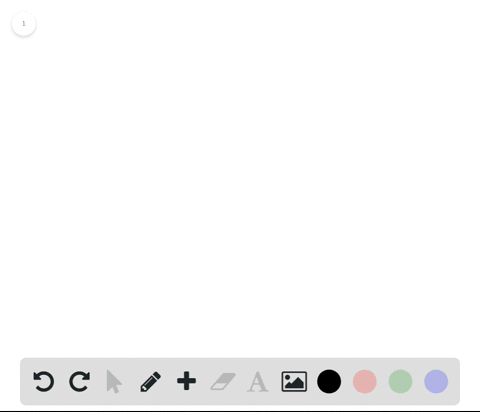Enroll in one of our FREE online STEM summer camps. Space is limited so join now!View Summer Courses### Comparing Functions and Limits If$f(2)=4$ can you…

00:47

Need more help? Fill out this quick form to get professional live tutoring.

Get live tutoring
Problem 64

Using the Definition of Limit The definition of limit on page 56 requires that $f$ is a function defined on
an open interval containing $c$ , except possibly at $c .$ Why is this requirement necessary?

Check back soon!

## Discussion

You must be signed in to discuss.

## Video Transcript

in this problem, we're asked why requirement that the function be defined on open toe containing see except possibly at sea is necessary for the definition of a limit. So we'll use the graft to the left to kind of understand a little bit more about that requirement. So to kind of sum it all up, we need the function to be defined around that value. See, because the limit is described by the behaviour of the function as Ex gets closer and closer to see it. So we need some open interval around that value to be defined, because within that interval is where we'll study the behaviour of the function. So even if you have seen is not to find the function is defined around see so we can study that. So let's study the example to the left and here will, let's see, be full to you, possibly two. No, If we look at our graph right here, we can see that limit as X approaches to is about 0.5 because the graph or the function values around too, from the left and the right are getting closer and closer to Sarah 0.5. This why value right here However you can see Fuck that the actual function value at two is not defined. But this doesn't effect the value of the limit. Hey!